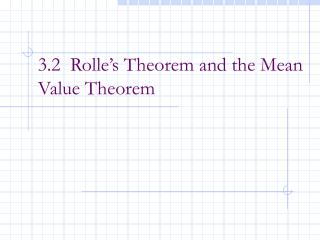DownloadDownload Presentation3.2 Rolle’s Theorem and the Mean Value Theorem

# 3.2 Rolle’s Theorem and the Mean Value Theorem

Download Presentation## 3.2 Rolle’s Theorem and the Mean Value Theorem

- - - - - - - - - - - - - - - - - - - - - - - - - - - E N D - - - - - - - - - - - - - - - - - - - - - - - - - - -
##### Presentation Transcript

1. 3.2 Rolle’s Theorem and the Mean Value Theorem

2. After this lesson, you should be able to: • Understand and use Rolle’s Theorem • Understand and use the Mean Value Theorem

3. f(a)=f(b) a b Rolle’s Theorem If you connect from f (a) to f (b) with a smooth curve, there will be at least one place where f’(c) = 0

4. Rolle’s Theorem Rolle's theorem is an important basic result about differentiable functions. Like many basic results in the calculus it seems very obvious. It just says that between any two points where the graph of the differentiable function f (x) cuts the horizontal line there must be a point where f '(x) = 0. The following picture illustrates the theorem.

5. Rolle’s Theorem height If two points at the same _______ are connected by a continuous, differentiable function, then there has to be ________ place between those two points where the derivative, or slope, is _____. at least one zero

6. Rolle’s Theorem If 1) f (x) is continuous on [a, b], 2) f (x) is differentiable on (a, b), and 3) f (a) = f (b) then there is at least one value of x on (a, b), call it c, such that f ’(c) = 0. f is continuous on [a, b] differentiable on (a, b) f(a) = f(b) a b

7. Example Example 1 ( f is continuous and differentiable) Since , then Rolle’s Theorem applies… then, x = –1 , x = 0, and x= 1

8. Rolle’s Theorem Does Rolle’s Theorem apply? If not, why not? If so, find the value of c. Example 2

9. Rolle’s Theorem Does Rolle’s Theorem apply? If not, why not? If so, find the value of c. Example 3

10. Example Example 4 (Graph the function over the interval on your calculator) continuous on [-1, 1] not differentiable at 0 not differentiable on (-1, 1) f(-1) = 1 = f(1) Rolle’s Theorem Does NOT apply since

11. Rolle’s Theorem Does Rolle’s Theorem apply? If not, why not? If so, find the value of c. Example 5

12. Note When working with Rolle’s make sure you1. State f(x)is continuous on [a, b] and differentiable on (a, b).2. Show that f(a) = f(b).3. State that there exists at least one x = cin (a, b)such that f ’(c) = 0. This theorem only guarantees the existence of an extrema in an open interval. It does not tell you how to find them or how many to expect. If YOU can not find such extrema, it does not mean that it can not be found. In most of cases, it is enough to know the existence of such extrema.

13. Mean Value Theorem- MVT The Mean Value Theorem is one of the most important theoretical tools in Calculus. It states that if f(x) is defined and continuous on the interval [a,b] and differentiable on (a,b), then there is at least one number c in the interval (a,b) (that is a<c<b) such that In other words, there exists a point in the interval (a,b) which has a horizontal tangent. In fact, the Mean Value Theorem can be stated also in terms of slopes. Indeed, the number is the slope of the line passing through (a, f(a)) and (b, f(b)). So the conclusion of the Mean Value Theorem states that there exists a point such that the tangent line is parallel to the line passing through (a, f(a)) and (b, f(b)).

14. (see Picture) The special case, when f(a) =f(b) is known as Rolle's Theorem. In this case, we have f '(c) =0.

15. Mean Value Theorem- MVT f If: f is continuous on [a, b], differentiable on (a, b) Then: there is a c in (a, b) such that a b

16. Example Example 6 (f is continuous and differentiable) MVT applies

17. “Peek”

18. Mean Value Theorem- MVT Note: ( ) The graph of f is rising a b

19. Mean Value Theorem- MVT Note: ( ) The graph of f is falling a b

20. Mean Value Theorem- MVT Note: ( ) The graph of f is level a b

21. Example Example 7 3

22. Finding a Tangent Line Example 8Find all values of c in the open interval (a, b) such that c= 1

23. Application of MVT Example 9When an object is removed from a furnace and placed in an environment with a constant temperature of 90o F, its core temperature is 1500o F. Five hours later the core temperature is 390o F. Explain why there must exist a time in the interval when the temperature is decreasing at a rate of 222o F per hour. Solution Let g(t) be the temperature of the object. Then g(0) = 1500, g(5) = 390 By MVT, there exists a time 0 <to <5, such that g’(to) = –222o F

24. Application of MVT Example 10Two bicyclists begin a race at 8:00 AM. They both finish the race 2 hours and 15 minutes later. Prove that at some time during the race, the bicyclists are traveling at the same velocity. Proof Let Si(t) be the distance function of bicyclist i (i = 1, 2), and let S(t) = S1(t)–S2(t). Then S(0) = S(2.25) = 0 By Rolle’s Theore, there exists a time 0 <to <2.25, such that S’(to) = S1’(to)–S2’(to) =v1(to)–v2(to)= 0 It produces v1(to)=v2(to)

25. Homework Section 3.2 page 172 #7-11 odd, 15, 31, 33, 37, 53-56